May 13, 2020

In the history of the internet, a collection of papers proposing PageRank has been influential, in particular, a 1998 paper by Sergey Brin and Lawrence Page, two graduate students, now better known as Google co-founders. They proposed an objective metric to order the results of a user's internet search. For those who don't remember, there was indeed a time when "junk results often wash[ed] out any results that a user is interested in," to quote the paper. Of course, search engines now operate in a competitive business world, and the algorithms that Google and other companies use to rank their search results currently are not public knowledge. But the concept of PageRank is now routinely applied beyond Google, not just to the internet, but to general data on graphs and networks. It serves as an automatic tool to rank the relative importance of parts of any internet-like giant network.

We shall view the web (internet) as a directed graph. Each internet location (a webpage) is a vertex of the graph. A hyperlink from one webpage to another is a directed edge of the graph. From this viewpoint, the central idea of Brin & Page was to exploit the "link structure of the web to calculate a quality ranking for each web page."

To introduce PageRank, we shall build on our previous discussion of Markov chains (from Gambler's Ruin), which was entirely from the statistical or probabilistic perspective. Below, we will connect to theorems of Perron and Frobenius, which are results that one might usually learn in a mathematics program. Of course, all of this helps us understand the effectiveness of PageRank, a topic that has entered the computer science curricula in recent decades. Taken together, we then have an example of propitious convergence of ideas from the distinct fields of computer science, mathematics, and statistics.

## Probability distributions on graphs¶

Throughout this discussion, we have in mind a directed graph with vertices $V_1, \ldots, V_N$, associated to a Markov chain with an $N \times N$ stochastic matrix $P = (p_{ij})$. We consider a random walker on this digraph, who we name $W$. The random walker $W$ is a "stochastic being". We cannot know $W$'s precise location on the graph; we only know that $W$'s location is determined by a probability distribution on the graph.

A probability vector is a vector $x \in \mathbb{R}^N$ whose entries $x_i$ satisfy $$0 \le x_i \le 1, \qquad \sum_{i=1}^N x_i =1.$$ Such a vector represents a probability distribution on the vertices of the graph. We may think of $x_i$ as the probability that the system is in state $V_i$. Alternatively, we may think of $x_i$ as the probability of finding the random walker $W$ at the digraph vertex $V_i.$

How does the probability of finding $W$ on the graph change when $W$ takes a step? Here is another way of asking the same question: if $x_i$ is the probability that the Markov chain is in state $V_i$, then what is the probability that the next state of the Markov chain is $V_j$? Since $V_j$ can be arrived at from $V_k$ with probability $p_{kj}$, and since the prior state was $V_k$ with probability $x_k$, we conclude that the answer should be the sum of $p_{kj} \times x_k$ over all the prior states $V_k$. In other words, the probability that the next state is $V_j$ equals $$\sum_{k=1}^N p_{kj} x_k,$$ which is the $j$th component of $P^t x$. This argument can be formalized to obtain the following basic result.

Theorem 1. The probability distribution of a random walk on a directed graph with stochastic matrix $P$ changes from $x$ to $P^t x$ in each step (where $P^t$ denotes the transpose of $P$).

Note that if $x$ is a probability vector and $P$ is a transition matrix, then $P^t x$ is guaranteed (exercise!) to come out as a probability vector.

## Stationary distributions¶

We have just seen that as the random walk progresses, an initial probability distribution $x$ changes as follows: $$x, \quad P^t x, \quad (P^t)^2 x, \quad (P^t)^3 x, \quad \ldots.$$ Suppose this sequence converges to a limiting vector $s$. Then that limit should obviously not change if one more $P^t$ is applied to it, i.e., it should satisfy $$P^t s = s.$$ Any probability vector $s$ satisfying $P^t s = s$ is called a stationary distribution, (or a stationary probability vector or an equilibrium) of the random walk. Notice that the stationary probability vector is always an eigenvector of $P^t$ associated to eigenvalue 1. Notice also that the limit, if it exists, is independent of the initial distribution $x$.

For the random walker $W$, if the limit of the above sequence exists, then the stationary distribution can be used to identify the vertices of the graph with a high probability of finding $W$ in the long run.

In :
import numpy as np
from numpy.linalg import eig, matrix_power, norm


#### Example A¶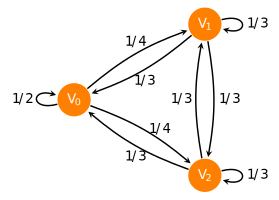In :
PA = np.array([[1/2,  1/4, 1/4],
[1/3,  1/3, 1/3],
[1/3,  1/3, 1/3]])


Does the sequence of probability distributions $x, \; P^t x, \; (P^t)^2 x, \; (P^t)^3 x, \, \ldots,$ converge for this Markov chain? To answer this, let's take the matrix powers $(P^t)^n$ and compute the Frobenius norm of the successive differences, $$\| (P^t)^{n+1} - (P^t)^n \|_F.$$ If this approaches 0, then we obtain a numerical indication of convergence.

In :
[norm(matrix_power(PA.T, n+1) - matrix_power(PA.T, n), 'fro') for n in range(1, 20)]

Out:
[0.1402709019216955,
0.023378483653615948,
0.0038964139422693537,
0.0006494023237115095,
0.00010823372061852081,
1.80389534364696e-05,
3.0064922394683963e-06,
5.010820398912459e-07,
8.351367329688623e-08,
1.3918945514670359e-08,
2.3198243477163972e-09,
3.86637279525466e-10,
6.44396235375308e-11,
1.073992260029489e-11,
1.789976112476022e-12,
2.9830188647232445e-13,
4.977570743895776e-14,
8.26743511340278e-15,
1.343782689223772e-15]

This indicates convergence. Let's check that the convergence actually occurs to a stationary distribution $s$ that is an eigenvector of $P^t$.

In :
ew, ev = eig(PA.T)
ew

Out:
array([0.16666667, 1.        , 0.        ])
In :
v = ev[:, abs(ew-1) < 1.e-14]; print(v)

[[0.68599434]
[0.51449576]
[0.51449576]]


This is the eigenvector corresponding to eigenvalue 1. In order to make this a probability distribution, let's normalize by the sum.

In :
sA = v / v.sum()
sA

Out:
array([[0.4],
[0.3],
[0.3]])

This is the stationary distribution $s$ for this example. To see that the same vector is obtained as the limit of $(P^t)^n$, we simply raise the matrix to a large power and examine the result:

In :
matrix_power(PA.T, 1000)

Out:
array([[0.4, 0.4, 0.4],
[0.3, 0.3, 0.3],
[0.3, 0.3, 0.3]])

The values of $s$ show that the random walker $W$ will, in the limit, be found in state $V_0$ at a higher probability ($0.4$) than the other two states ($0.3$).

#### Example B¶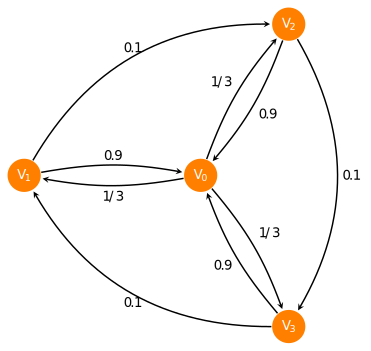In :
PB = np.array([[0,  1/3, 1/3, 1/3],
[0.9, 0,   0,  0.1],
[0.9, 0.1, 0,    0],
[0.9, 0,  0.1,   0]])

In :
ew, ev = eig(PB.T); print(ew)

[-0.9 +0.j          1.  +0.j         -0.05+0.08660254j -0.05-0.08660254j]

In :
# stationary distribution:
v = ev[:, abs(ew-1) < 1.e-14];
sB = v.real / sum(v.real); print(sB)

[[0.47368421]
[0.1754386 ]
[0.1754386 ]
[0.1754386 ]]


In this example, there is convergence of the powers to the stationary distribution, but it is slower than Example A. We find this out by taking higher powers than before:

In :
[norm(matrix_power(PB.T, n) - sB, 'fro') for n in range(300, 305)]

Out:
[2.0819420081737047e-14,
1.9224558387957245e-14,
1.6814381771214046e-14,
1.558237665379239e-14,
1.3619335994971806e-14]

#### Example C¶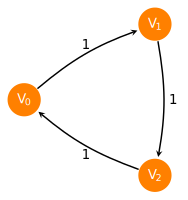In :
PC = np.array([[0,  1,  0],
[0,  0,  1],
[1,  0,  0]])

In :
ew, ev = eig(PC.T); print(ew)

[-0.5+0.8660254j -0.5-0.8660254j  1. +0.j       ]

In :
# stationary distribution:
v = ev[:, abs(ew-1) < 1.e-14].real; sC = v/v.sum(); print(sC)

[[0.33333333]
[0.33333333]
[0.33333333]]


In this example, we do not see convergence of the powers $P^t$ to the above stationary distribution. There seems to be no convergence to anything:

In :
[norm(matrix_power(PC.T, n+1) - matrix_power(PC.T, n)) for n in range(100, 105)]

Out:
[2.449489742783178,
2.449489742783178,
2.449489742783178,
2.449489742783178,
2.449489742783178]

These numbers clearly do not seem to be approaching zero, a sign of non-convergence. In fact, the transition matrix here is such that all its powers cycle between three matrices $P^t, (P^t)^2$ and $(P^t)^3$, thus preventing convergence!

In :
[print('The %dth power:\n'%i, matrix_power(PC.T, i)) for i in range(300, 306)];

The 300th power:
[[1 0 0]
[0 1 0]
[0 0 1]]
The 301th power:
[[0 0 1]
[1 0 0]
[0 1 0]]
The 302th power:
[[0 1 0]
[0 0 1]
[1 0 0]]
The 303th power:
[[1 0 0]
[0 1 0]
[0 0 1]]
The 304th power:
[[0 0 1]
[1 0 0]
[0 1 0]]
The 305th power:
[[0 1 0]
[0 0 1]
[1 0 0]]


#### Summary of the three examples:¶

Example A Example B Example CConvergent: $\lim_{n\to\infty}(P^t)^n x =s$ Convergent: $\lim_{n\to\infty}(P^t)^n x =s$ Not convergent:
$\lim_{n\to\infty}(P^t)^n$ doesn't exist
Stationary distribution: Stationary distribution: Stationary distribution:
$s= \begin{bmatrix} 0.4 \\ 0.3\\ 0.3\end{bmatrix}$ $s = \begin{bmatrix} 0.474 \\ 0.175 \\ 0.175\\ 0.175 \end{bmatrix}$ $s= \begin{bmatrix} 1/3 \\ 1/3 \\ 1/3 \end{bmatrix}$

Note how associating the values of $s_i$ to vertex $V_i$ produces something that matches our intuition on where to find the random walker in the long run. The convergence in Example A is a consequence of Perron's theorem that we discuss next.

## Perron's theorem¶

The following celebrated result in linear algebra was proved by Oskar Perron (about 90 years before Brin & Page's paper). Research papers continue to be written on subjects surrounding the theorem. The theorem applies to any positive matrix: a square matrix is called a positive matrix if all its entries are positive.

Theorem 2. The following statements hold for any positive matrix $A$.

• There is a positive real number $\mu$ that is an eigenvalue of $A$ such that any other eigenvalue $\lambda$ of $A$ is smaller in absolute value: $|\lambda | < \mu$. (This $\mu$ is called the dominant eigenvalue of $A$.)

• The eigenspace of the eigenvalue $\mu$ is one-dimensional and contains an eigenvector $v$ whose entries $v_i$ are all positive.

• The limit $\displaystyle{ \lim_{n \to \infty} \frac{1}{\mu^n} A^n }$ exists and equals a matrix whose columns are all scalar multiples of $v$.

#### Graphical illustration¶

If you have never seen Theorem 1 before, you might be mystified how so many strong statements can be concluded simply from the positivity assumption. I'd like to give you an idea of the reasoning that leads to these statements, without writing out a formal proof, through the following simple example of a $2 \times 2$ positive matrix.

In :
A = np.array([[0.1, 0.9],
[0.6, 0.4]])
ew, ev = eig(A)
ew

Out:
array([-0.5,  1. ])

We see that the dominant eigenvalue is 1 in this case. To get an idea of why $A^n$ converges, as claimed in the theorem, see what happens when we multiply $A$ by $A$ in terms of the first and second columns ($A_0$ and $A_1$) of $A = [A_0, A_1]$: $$A^2 = A [ A_0, A_1] = [A A_0, A A_1]$$ When $A$ is multiplied by a positive vector the result is a linear combination of the columns of $A$ with positive combination coefficients. This is best seen using pictures. To this end, we define a function below that plots the columns of $A$ (as two thick arrows) and the region in between (a two-dimensional cone) using criss-cross lines.

Using it we see what happens to the cone region under repeated application of $A$.

In :
import matplotlib.pyplot as plt
%matplotlib inline

def plotcone(A0, A1, xlim=(0,1.1), ylim=(0,1.), matlabel='$A$', tt='Illustration of convergence of $A^n$'):
t = np.linspace(0, 3, num=100)
gridline0 = t[:, np.newaxis] * A0
gridline1 = t[:, np.newaxis] * A1
fig = plt.figure(); ax = plt.gca()
for i in range(20):
ax.plot(gridline0[:, 0], gridline0[:, 1], 'b')
ax.plot(gridline1[:, 0], gridline1[:, 1], 'r')
gridline0 += (1/5) * A1
gridline1 += (1/5) * A0
ax.set_xlim(xlim);  ax.set_ylim(ylim)
ax.set_title(tt)
a0 = ax.arrow(0, 0, A0, A0, width=0.05, color='blue', alpha=0.3)
a1 = ax.arrow(0, 0, A1, A1, width=0.05, color='red', alpha=0.3)
plt.legend((a0, a1), ('First column vector of '+matlabel, 'Second column vector of '+matlabel), loc='lower right');

In :
M = A.copy()
for i in range(5):  # plot the cone between columns for each matrix power
A0 = M[:, 0]
A1 = M[:, 1]
plotcone(A0, A1, matlabel='$A^'+str(i+1)+'$')
M = M @ A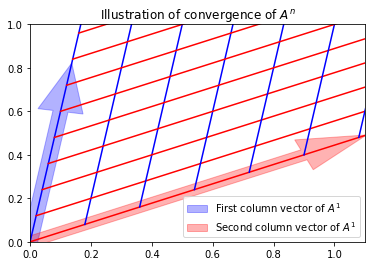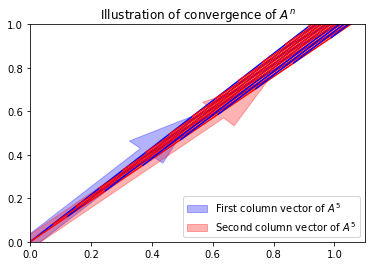As you can see, repeated application of $A$ eventually squeezes the cone region to a linear region. The vectors in the boundary of the region getting squeezed are the columns of $A^n$ as $n \to \infty$, so you have just seen a pictorial illustration of existence of the limit of $A^n$, and also of the theorem's claim that in the limit, the columns become multiples of a single vector. Moreover, the limiting linear region in the figure should remain unaltered under further applications of $A$, so it must represent an eigenspace of $A$. Note also that all of this happens in the positive quadrant of the plane, so obviously the squeezed in line is the span of a vector $v$ with positive entries, so this should be the positive eigenvector mentioned in the theorem.

This squeezing phenomena happens because $A$ has positive entries. If $A$ had negative entries, the region between its column vectors need not get so squeezed and can dance all over the place preventing convergence of its powers. Considering another matrix, also with dominant eigenvalue 1, but now with a negative entry, you get the picture:

In :
B = np.array([[0.1, -1.6],   # change sign of one entry of A to get B
[0.6, 0.4]])
plotcone(B[:,0], B[:,1], xlim=(-2.1, 0.5), ylim=(-1.1, 1.1), matlabel='$B$', tt='Noncovergence')
B = B @ B
plotcone(B[:,0], B[:,1], xlim=(-2.1, 0.5), ylim=(-1.1, 1.1), matlabel='$B^2$', tt='Noncovergence')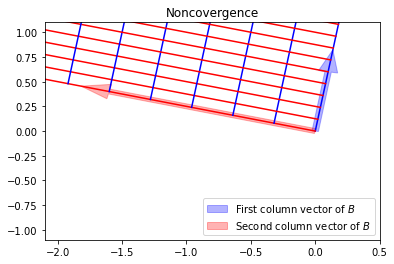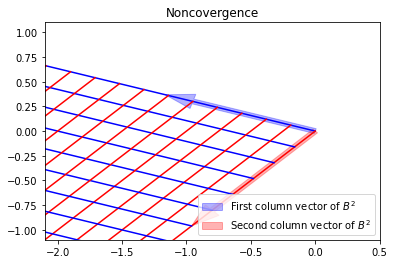Any overlaps between the cones disappear as you take further powers.

This completes our graphical illustration of the connection between positivity of entries, the convergence of matrix powers, and the resulting capture of a positive eigenvector by successively squeezing cones. In the next subsection, where we apply Perron's theorem to transition matrices, we will be more rigorous, and yet, use nothing more than what you already know from your linear algebra prerequisites.

#### Application to stochastic matrices¶

In this subsection, we consider a Markov chain whose transition matrix $P=(p_{ij})$ has positive entries. Accordingly, there is a dominant positive eigenvalue $\mu$ and corresponding eigenvector $v$ with positive components: $P v = \mu v$. Normalizing $v$ such that its maximum entry $v_i$ is one, the $i$th equation of the system $Pv = \mu v$ reads as $$\sum_{j=1}^N p_{ij} v_j = \mu v_i = \mu.$$ We also have $$\sum_{j=1}^N p_{ij} v_j \le \sum_{j=1}^N p_{ij} = 1.$$ Putting these together, we conclude that the dominant eigenvalue satisfies $\mu \le 1$. But any transition matrix $P$ always has $1$ as an eigenvalue. This is because of the fact that its rows sums are one $$\sum_{j=1}^N p_{ij} = 1$$ can be rewritten in matrix terms as $$P \begin{bmatrix} 1 \\ \vdots \\ 1 \end{bmatrix} = \begin{bmatrix} 1 \\ \vdots \\ 1 \end{bmatrix}$$ Therefore $\mu$ must be 1. Let's highlight this conclusion:

• $\mu=1$ is the dominant eigenvalue of any positive transition matrix $P$ and the corresponding eigenvector is the vector whose entries are all ones.

Of course, Perron's theorem applies to both $P$ and $P^t$, since both are positive matrices. Since the eigenvalues of $P$ and $P^t$ are the same, we can further say this:

• $\mu=1$ is also the dominant eigenvalue of $P^t$ (but the eigenvector corresponding to eigenvalue 1 may be different for $P^t$ and $P$).

The theorem also tells us that the limit of $(P^t)^n$ exists. Relating to our discussion of stationary distributions, we conclude:

• The sequence of probability distributions of a random walk $$x, \quad P^t x, \quad (P^t)^2 x, \quad (P^t)^3 x, \quad \ldots$$ always converges for Markov chains with $p_{ij}>0$ and the limit $s$ is independent of the initial distribution $x$.

The theorem also tells us that the limit of $P^n$ exists, and moreover, the limit matrix must have columns that are scalar multiples of the eigenvector of the dominant eigenvalue: in other words, the columns of $\lim_{n\to \infty} P^n$ must be scalar multiples of the vector of ones. On the other hand, the limit of $(P^t)^n = (P^n)^t$ must have columns that are multiples of the eigenvector $s$ satisfying $P^t s = s,$ which we previously called the stationary distribution. Thus, having pinned down the rows and the columns, we make the following conclusion:

• The limit of $P^n$ as $n \to \infty$ takes the following form $$\lim_{n\to \infty} P^n = \begin{bmatrix} s_1 & s_2 & \ldots & s_N \\ s_1 & s_2 & \ldots & s_N \\ \vdots & \vdots & & \vdots \\ s_1 & s_2 & \ldots & s_N \end{bmatrix}.$$

Example A has a positive transition matrix. It provides an instance where all the previous statements can be verified. For example, $\lim_{n \to \infty} P^n$ is approximated by the matrix below which reveals the above pattern of stationary distributions in each row.

In :
matrix_power(PA, 1000)   # P^1000 for Example A

Out:
array([[0.4, 0.3, 0.3],
[0.4, 0.3, 0.3],
[0.4, 0.3, 0.3]])
In :
sA                       # the stationary distribution for Example A

Out:
array([[0.4],
[0.3],
[0.3]])

## PageRank¶

We shall now define the PageRank of vertices on an (unweighted) directed graph with $N$ vertices.

1. First, set $a_{ij} = 1$ if there is an edge from vertex $v_i$ to $v_j$ in the graph and set $a_{ij}=0$ otherwise.

2. Next, let $$m_i = \sum_{k=1}^N a_{ik}.$$ If $m_i$ is 0, then the $i$th vertex is a dangling node (which has no outgoing edges). Define w_{ij} = \left\{ \begin{aligned} & \frac{a_{ij}}{m_i} && \text{ if } m_i > 0, \\ & \frac 1 N && \text{ if } m_i = 0. \end{aligned} \right. These may be thought of as weights on a directed edge from $v_i$ to $v_j$ if the edge exists (if not, the weight is zero). The weight $w_{ij}$ may also be viewed as providing equal probabilities to all outgoing edges from $v_i$.

1. Now that we have a weighted directed graph, we may associate it to a Markov chain, setting transition probabilities to $w_{ij}$, but hold on: if we do so, a random walker $W$ on the graph can get stuck in vertices with no outgoing edges or in cycles within the graph. (This is certain to happen on graphs as complex as the internet.) To avoid this situation, one sets a restart probability $0 < r \ll 1$ with which $W$ is allowed to jump from one vertex to any other vertex in the graph. (Page called $1-r$ the damping factor.)

2. Finally, set the Markov chain transition probabilities by

$$p_{ij} = \frac{r}{N} + (1-r) w_{ij}.$$

The PageRank of a vertex is defined to be the value of the stationary probability distribution at that vertex obtained using the above $p_{ij}$ as the transition probabilities.

Note that the transition matrix $(p_{ij})$ defined above is a positive matrix. Hence, due to Perron's theorem, and our prior discussion on its application to stochastic matrices, the limit of the probability distributions
$$x, \quad P^t x, \quad (P^t)^2 x, \quad (P^t)^3 x, \quad \ldots$$ exists, is equal to the stationary probability distribution, which we have just decided to call PageRank. In particular, PageRank is independent of the starting distribution $x$ of the random walk. Furthermore, we may also arrive at the interpretation of the PageRank of a graph vertex as the limiting probability that a relentless random walker visits that vertex.

Here is a simple implementation for small graphs using the same notation ($a_{ij}, w_{ij}, p_{ij}$) as above. (Think about what would need to change for a giant graph like the internet. We'll consider big graphs in later exercises.)

In :
def pagerank(a, r):
""" Return pagerank based on adjacency matrix "a" (square matrix
of 0s or 1s) and given restart probability "r". Use only for small
dense numpy matrices a. """

m = a.sum(axis=1)
dangling = (m==0)
m[dangling] = 1

w = (1 / m[:, np.newaxis]) * a
w[dangling, :] = 1 / a.shape

p = (1-r) * w  +  (r / a.shape)
ew, ev = eig(p.T)
s = ev[:, abs(ew-1) < 1e-15].real
return  s / s.sum()


Let's quickly consider a small example to illustrate PageRank.

#### Example D¶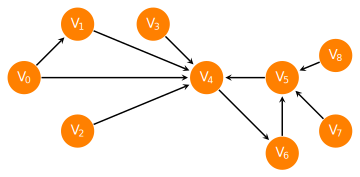In :
#              0  1  2  3  4  5  6  7  8   (Adjacency Matrix of the above graph)
A = np.array([[0, 1, 0, 0, 1, 0, 0, 0, 0],  # 0
[0, 0, 0, 0, 1, 0, 0, 0, 0],  # 1
[0, 0, 0, 0, 1, 0, 0, 0, 0],  # 2
[0, 0, 0, 0, 1, 0, 0, 0, 0],  # 3
[0, 0, 0, 0, 0, 0, 1, 0, 0],  # 4
[0, 0, 0, 0, 1, 0, 0, 0, 0],  # 5
[0, 0, 0, 0, 0, 1, 0, 0, 0],  # 6
[0, 0, 0, 0, 0, 1, 0, 0, 0],  # 7
[0, 0, 0, 0, 0, 1, 0, 0, 0]]) # 8

In :
pagerank(A, 0.1)

Out:
array([[0.01111111],
[0.01611111],
[0.01111111],
[0.01111111],
[0.32328823],
[0.30297458],
[0.30207052],
[0.01111111],
[0.01111111]])

Notice from the output that $V_4$ is ranked highest. A vertex to which many other vertices points to usually get a higher PageRank. Notice also how a vertex to which a highly ranked vertex points to inherits a high PageRank: this is the case with vertex $V_6$. Vertex $V_5$ is also highly ranked because it has its own cluster of vertices ($V_6, V_7, V_8$) pointing to it which included one highly ranked vertex $V_6$.

It is instructive to look at how PageRank changes as the restart probability is decreased to 0:

In :
pagerank(A, 0.01)

Out:
array([[0.00111111],
[0.00166111],
[0.00111111],
[0.00111111],
[0.33239996],
[0.33019631],
[0.33018707],
[0.00111111],
[0.00111111]])
In :
pagerank(A, 0.0)

Out:
array([[-0.        ],
[-0.        ],
[-0.        ],
[-0.        ],
[ 0.33333333],
[ 0.33333333],
[ 0.33333333],
[-0.        ],
[-0.        ]])

This identifies the cycle where the random walker would end up if it were not for the restart mechanism.

#### On internet search results¶

As I mentioned above, PageRank was proposed specifically to order the world wide web. In view of our previous discussion, when applied to the giant graph of the internet, the PageRank of a webpage can be interpreted as the steady state probability that a random web surfer, following hyperlinks from page to page (with infinite dedication and with no topical preference), is at that webpage.

When a user types in a search query, a search engine must first be able to mine all the webpages relevant to the query from its database. (PageRank does not help with this task.) Then, it must present these pages in some order to user. If the search engine has already computed a ranking of relative importance of each webpage, then it can present the results to the user according to that ranking. This is where PageRank helps. It does require the search engine to solve for a giant eigenvector (with billions of entries) to compute PageRank on the entire world wide web. The results of this computation (which cannot be done in real time as the user searches) are stored by the search engine. The stored ranking is then used to order the results presented to the user. There are reports that Google does this a couple of times a year (but I don't know how to verify this).

## Perron-Frobenius theorem¶

Georg Frobenius generalized Perron's theorem to nonnegative matrices. The key discovery of Frobenius was that although many of the nice properties of positive matrices fail to hold for general non-negative matrices, they continue to hold for non-negative matrices whose directed graph exhibits a "nice" property. Recall that the directed graph of an $N \times N$ matrix $A = (a_{ij})$ is a graph with vertices $1, 2, \ldots, N$ which has a directed edge from vertex $i$ to vertex $j$ whenever $a_{ij}$ is nonzero. The "nice" property is the following.

A square matrix is called irreducible if its directed graph is such that there is a path made of directed edges from any vertex to any other vertex.

Theorem 3. (Perron-Frobenius) The following statements hold for any irreducible $N \times N$ matrix $A=(a_{ij})$ whose entries satisfy $a_{ij}\ge 0$ are all non-negative.

• The maximum $\mu$ of the absolute value of all eigenvalues of $A$ is an eigenvalue of $A$.

• The eigenspace of the eigenvalue $\mu$ is one-dimensional and contains an eigenvector $v$ whose entries $v_i$ are all positive.

• The limit $\displaystyle{ \lim_{k \to \infty} \frac 1 k \sum_{n=0}^{k-1} \frac{1}{\mu^n} A^n }$ exists and equals a matrix whose columns are all scalar multiples of $v$.

Note the main differences in Theorem 3 in comparison to Theorem 2:

• Unlike positive matrices, now there might be more than one eigenvalue whose absolute value is $\mu$.

• Unlike positive matrices, we can no longer assert that limit $A^n/\mu^n$ exists, only that the limit of averages of $A^n/\mu^n$ exists.

#### Reconsider Examples B & C¶

Example B Example C